annotation

Create annotations

Description

example

annotation(lineType,x,y) creates a line or arrow annotation extending between two points in the current figure. Specify lineType as 'line', 'arrow', 'doublearrow', or 'textarrow'. Specify x and y as two-element vectors of the form [x_begin x_end] and [y_begin y_end], respectively.

annotation(lineType) creates the annotation in the default position between the points (0.3,0.3) and (0.4,0.4).

example

annotation(shapeType,dim) creates a rectangle, ellipse, or text box annotation with a particular size and location in the current figure. Specify shapeType as 'rectangle', 'ellipse', or 'textbox'. Specify dim as a four-element vector of the form [x y w h]. The x and y elements determine the position and the w and h elements determine the size.

annotation(shapeType) creates the annotation in the default position so that the lower left corner is at (0.3,0.3) and the width and height are both 0.1.

example

annotation(___,Name,Value) creates the annotation and specifies properties as name-value pair arguments. Different types of annotations support different properties. You can specify properties with any of the input argument combinations in the previous syntaxes.

annotation(container,___) creates the annotation in the figure, uipanel, or uitab specified by container, instead of in the current figure.

example

an = annotation(___) returns the annotation object. The type of object returned depends on first input argument. Use an to modify properties of the object after it is created. You can specify an output argument with any of the previous syntaxes.

Examples

collapse all

Create a simple line plot and add a text arrow to the figure. Specify the text arrow location in normalized figure coordinates, starting at the point (0.3,0.6) and ending at (0.5,0.5). Specify the text description by setting the String property.

figure
plot(1:10)
x = [0.3 0.5];
y = [0.6 0.5];
annotation('textarrow',x,y,'String','y = x ')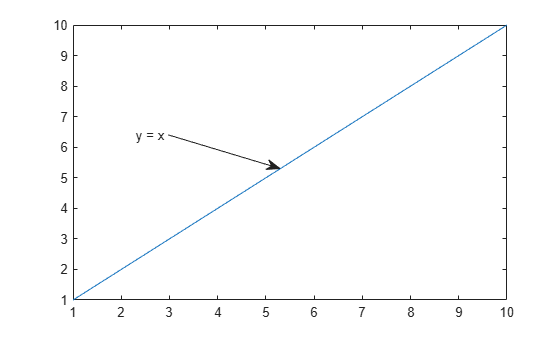Create a simple line plot and add a text box annotation to the figure. Specify the text description by setting the String property. Force the box to fit tightly around the text by setting the FitBoxToText property to 'on'.

figure
plot(1:10)
dim = [.2 .5 .3 .3];
str = 'Straight Line Plot from 1 to 10';
annotation('textbox',dim,'String',str,'FitBoxToText','on');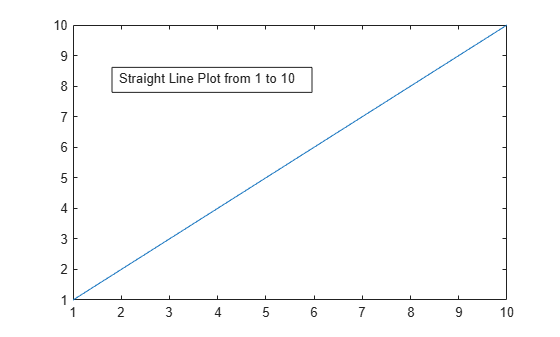Create a text box annotation without setting the FitBoxToText property. The text box uses the specified width and height and wraps text as needed.

figure
plot(1:10)
dim = [.2 .5 .3 .3];
str = 'Straight Line Plot from 1 to 10';
annotation('textbox',dim,'String',str)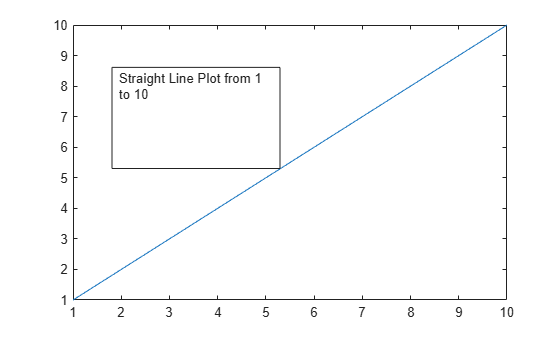Create a text box annotation with multiline text by setting the String property to a cell array. Each element of the cell array displays on a separate line. Force the box to fit tightly around the text by setting the FitBoxToText property to 'on'.

figure
plot(1:10)
dim = [0.2 0.5 0.3 0.3];
str = {'Straight Line Plot','from 1 to 10'};
annotation('textbox',dim,'String',str,'FitBoxToText','on');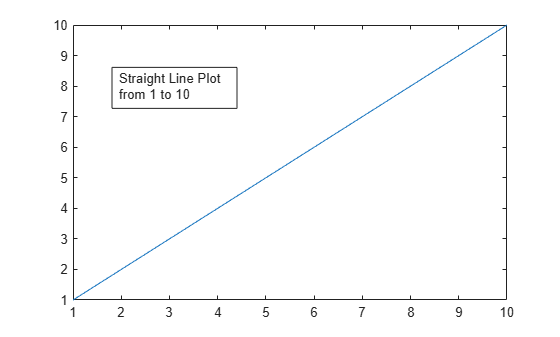Create a stem plot and add a rectangle annotation to the figure. Change the color of the rectangle outline by specifying the Color property.

figure
data = [2 4 6 7 8 7 5 2];
stem(data)
dim = [.3 .68 .2 .2];
annotation('rectangle',dim,'Color','red')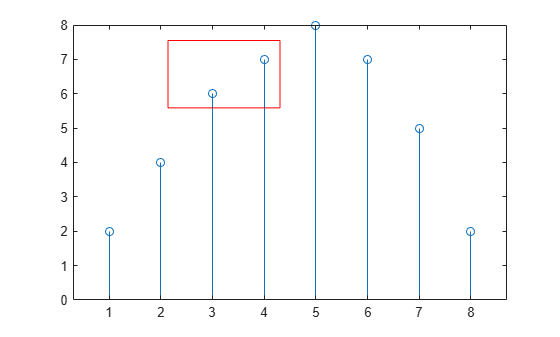Add a second rectangle annotation to the figure. Specify the fill color by setting the FaceColor property. Add transparency by setting the FaceAlpha property to a value between 0 (completely transparent) and 1 (completely opaque).

dim2 = [.74 .56 .1 .1];
annotation('rectangle',dim2,'FaceColor','blue','FaceAlpha',.2)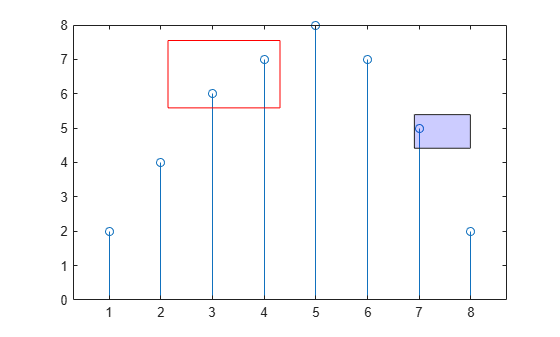Create a simple line plot and add an ellipse annotation to the figure. Specify dim as the size and location of the smallest rectangle that encloses the ellipse.

figure
x = linspace(-4,4);
y = x.^3 - 12*x;
plot(x,y)

dim = [.2 .74 .25 .15];
annotation('ellipse',dim)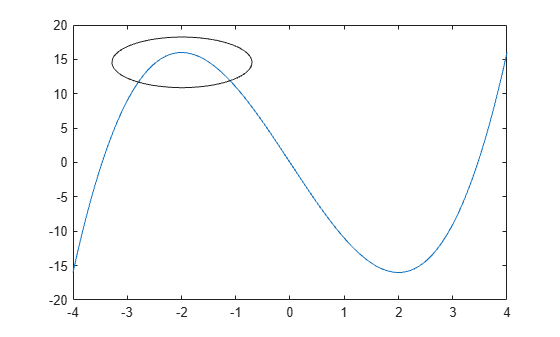Draw a red rectangle using the same dimensions to show how the ellipse fills the rectangular area.

annotation('rectangle',dim,'Color','red')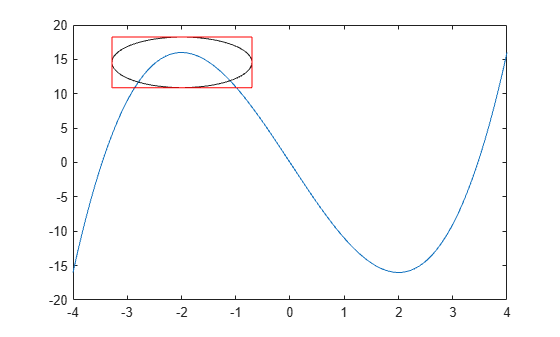Create a simple line plot. Then, add a bent arrow to the graph by combining a line and an arrow annotation.

figure
plot(1:10)

xl = [.3 .3];
yl = [.3 .4];
annotation('line',xl,yl)

xa = [.3 .4];
ya = [.4 .4];
annotation('arrow',xa,ya)Add a text arrow to a figure and return the annotation text arrow object, a.

figure
plot(1:10)
x = [0.3,0.5];
y = [0.6,0.5];
a = annotation('textarrow',x,y,'String','y = x ');Modify properties of the annotation text arrow using a. For example, change the color to red and the font size to 14 points.

a.Color = 'red';
a.FontSize = 14;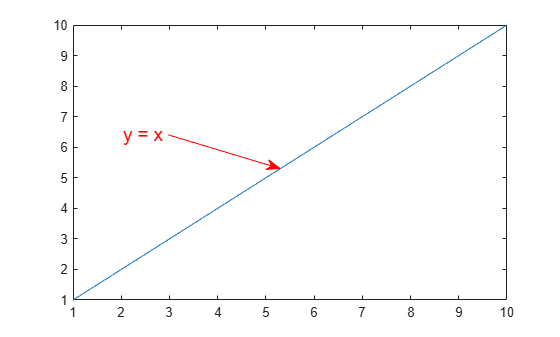See the annotation property pages for a list of properties for each type of annotation.

Input Arguments

collapse all

Type of line annotation, specified as one of these values.

ValueType of ObjectExample
'line'Annotation lineannotation('line',[.1 .2],[.1 .2])
'arrow'Annotation arrowannotation('arrow',[.1 .2],[.1 .2])
'doublearrow'Annotation double arrowannotation('doublearrow',[.1 .2],[.1 .2])
'textarrow'

Annotation text arrow. To add text to the tail end of the text arrow, use the String property.

annotation('textarrow',[.1 .2],[.1 .2],'String','my text')

Type of shape annotation, specified as one of these values.

ValueType of ObjectExample
'rectangle'Annotation rectangleannotation('rectangle',[.2 .3 .4 .5])
'ellipse'Annotation ellipseannotation('ellipse',[.2 .3 .4 .5])
'textbox'

Annotation text box. To specify the text, set the String property. To automatically adjust the dimensions of the box to fit closely around the text, set the FitBoxToText property to 'on'.

annotation('textbox',[.2 .3 .4 .5],'String','my text','FitBoxToText','on')

Beginning and ending x-coordinates, specified as a two-element vector of the form [x_begin x_end]. Together the x and y input arguments determine the endpoints of the line, arrow, double arrow, or text arrow annotation. The annotation extends from the point (x_begin, y_begin) to (x_end, y_end).

By default, the units are normalized to the figure. The lower left corner of the figure maps to (0,0) and the upper right corner maps to (1,1). To change the units, use the Units property.

Example: x = [.3 .5]

Beginning and ending y-coordinates, specified as a two-element vector of the form [y_begin y_end]. Together the x and y input arguments determine the endpoints of the line, arrow, double arrow, or text arrow annotation. The annotation extends from the point (x_begin, y_begin) to (x_end, y_end).

By default, the units are normalized to the figure. The lower left corner of the figure maps to (0,0) and the upper right corner maps to (1,1). To change the units, use the Units property.

Example: y = [.3 .5]

Size and location, specified as a four-element vector of the form [x y w h]. The first two elements specify the coordinates of the lower left corner of the text box, rectangle, or ellipse with respect to the lower left corner of the figure. The second two elements specify the width and height of the annotation, respectively.

If you are creating an ellipse, then dim is the size and location of the smallest rectangle that encloses the ellipse.

By default, the units are normalized to the figure. The lower left corner of the figure maps to (0,0) and the upper right corner maps to (1,1). To change the units, use the Units property

Example: dim = [.3 .4 .5 .6]

Target for annotation, specified as a figure, uipanel, or uitab object. For example, to add an annotation to a specific figure, specify the figure object as the first input argument to the function.

f = figure;
annotation(f,'line',[.1 .2],[.2 .3])

Name-Value Arguments

Specify optional comma-separated pairs of Name,Value arguments. Name is the argument name and Value is the corresponding value. Name must appear inside quotes. You can specify several name and value pair arguments in any order as Name1,Value1,...,NameN,ValueN.

Example: annotation('rectangle',[.5 .5 .1 .1],'EdgeColor','r') creates a rectangle annotation with a red outline.

Each type of annotation object supports a different set of properties. For a full list of properties and descriptions for each type, see the associated property page.

Output Arguments

collapse all

Annotation object, returned as a scalar. The type of annotation object returned depends on the first input argument.

First Input ArgumentType of Object ReturnedProperty Page
'line'LineLine Properties
'arrow'ArrowArrow Properties
'doublearrow'Double arrowDoubleEndArrow Properties
'textarrow'Text arrowTextArrow Properties
'textbox'Text boxTextBox Properties
'rectangle'RectangleRectangle Properties
'ellipse'EllipseEllipse Properties